### Latticedamage2d

This is a damage lattice material used together with latticedamage2d elements. It uses a scalar damage relationship of the form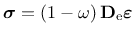(233)

where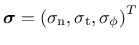is a vector of tractions and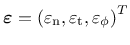is a vector of strains obtained from displacement jumps smeared over the element length. Furthermore,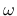is the damage variable varying from 0 (undamaged) to 1 (fully damaged). Also,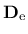is the elastic stiffness matrix which is based on the elastic modulus of the lattice material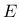, and a parameter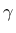which is the ratio of the modulus of the shear and normal direction. The strength envelope is elliptic (Figure 13) and determined by three parameters,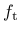,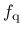and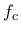. The evolution of the damage variableis controlled by normal stress-normal crack opening law. The three possible laws are linear, bilinear and exponential (Figure 14).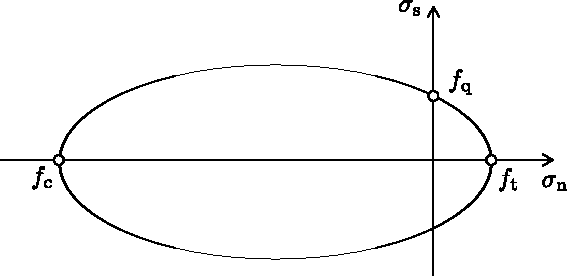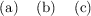The model parameters are summarised in Tab. 51.

Table 51: Scalar damage model for 2d lattice elements - summary.
 Description Saclar damage model for lattice2d Record Format latticedamage2d (in) # d(rn) # talpha(rn) # e(rn) # a1(rn) # a2(rn) # e0(rn) # coh(rn) # ec(rn) # stype(rn) # wf(rn) # wf1(rn) # Parameters - material number - d material density - talpha Thermal exansion coefficient - e normal modulus of lattice material - a1 ratio of shear and normal modulus - a2 ratio of rotational and normal modulus. Optional parameter. Default is 1. - e0 strain at tensile strength: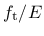- coh ratio of shear and tensile strength: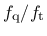- ec ratio of compressive and tensile strength: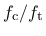- stype softening types: 1-linear, 2-bilinear and 3-exponential - wf displacement threshold related to fracture energy used in all three softening types. Supported modes 2dlattice

Borek Patzak
2019-03-19